# Application of OP-Amp as Summing amplifier, Integrator and Differentiator

#### Summing Amplifier

The output voltage of a summing amplifier is proportional to the negative of the algebraic sum of its input voltages. Hence, the name summing amplifier. A summing amplifier is an inverted OP-Amp that can accept two or more inputs.

Fig.1 shows a three-input summing amplifier.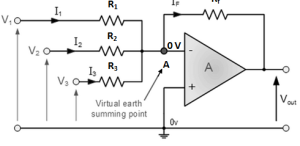Fig.1

Three voltages V1, V2 and V3 are applied to the inputs and produce currents I1, I2 and I3.

The inverting input of the OP-Amp is at virtual ground (0 V) and there is no current to the input.

So, the three input currents I1, I2 and I3 combine at the summing point A and form the total current If which goes through Rf as shown in fig.1.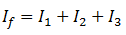When all the three inputs are applied, the output voltage is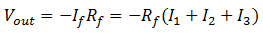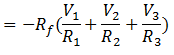If R1=R2=R3=R, then, we have,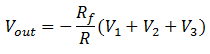Thus the output voltage is proportional to the algebraic sum of the input voltages.

If Rf =R1=R2=R3=R, then, we haveThus, when the gain of summing amplifier is unity, the output voltage is the algebraic sum of the input voltages.

#### Application of Summing Amplifier

There are a number of applications of summing amplifiers. Here we will discuss the following two applications:

1. As averaging amplifier
2. As Subtractor

#### 1.  As Averaging Amplifier

By using the proper input and feedback resistor values, a summing amplifier can be designed to provide an output voltage that is equal to the average of input voltages.

A summing amplifier will act as an averaging amplifier when both of the following conditions are met:

• All input resistors (R1, R2 and so on ) are equal in value.
• The ratio of any input resistor to the feedback resistor is equal to the number of input circuits.

Fig.2 shows the circuit of averaging amplifier.

Fig.2

Here all input resistors are equal in value (3 KΩ). If we take the ratio of any input resistor to the feedback resistor, we get 3 kΩ/1 kΩ =3. This is equal to the number of inputs to the circuit.

Referring to fig.1, the output voltage is given by:

Now,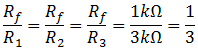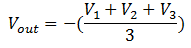Note that Vout is equal to the average of the three inputs. The negative sign shows the phase reversal.

#### 2.  As Subtractor

A summing amplifier can be used to provide an output voltage that is equal to the difference of two  voltages.

Such a circuit is called a Subtractor and is shown if fig.3.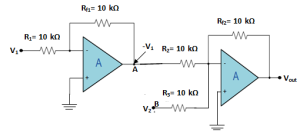Fig.3

As we can see, this circuit will provide an output voltage that is equal to the difference between V1 and V2.

The voltage V1 is applied to a standard inverting amplifier that has unity gain.

Because of this, the output from the inverting amplifier will be equal to –V1.

This output is then applied to the summing amplifier, also having unity gain along with V2.

Thus output from second OP-Amp is given by: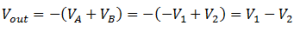The gain of the second stage in the Subtractor can be varied to provide an output that is proportional to the difference between the input voltages.

#### OP-Amp Integrator

An integrator is a circuit that performs integration of the input signal. The most important application of an integrator is to produce a ramp output voltage.

Fig.4 shows the circuit of an OP-Amp integrator.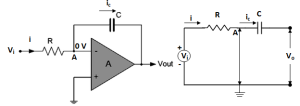Fig.4(i)                                                                    Fig.4 (ii)
When a signal is applied to the input of this circuit, the output-signal waveform will be the integration of input-signal waveform.It consists of an OP-Amp, input resistor R and feedback capacitor C.

#### Circuit Analysis

Since point A in fig.4(i) is at virtual ground, the virtual ground equivalent circuit of operational integrator will be as shown in fig.4 (ii).

Because of virtual ground and infinite impedance of the OP-Amp, all of the input current flows through the capacitor i.e.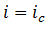Now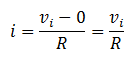Also voltage across capacitor isSo,Since,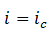So,Or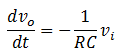To find out the output voltage, we integrate both sides of the above equation to get,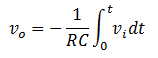This equation shows that the output is the integral of the input with an inversion and scale multiplier of 1/RC.

#### Output Voltage

If a fixed voltage is applied to the input of an integrator, the output voltage grows over a period of time, providing a ramp voltage.

The output ramp voltage is opposite in polarity to the input voltage and is multiplied by a factor 1//RC.

#### OP-Amp Differentiator

A differentiator is a circuit that performs differentiation of the input signal. That means, a differentiator produces an output voltage that is proportional to the rate of change of the input voltage.

Its important application is to produce a rectangular output from a ramp input.

Fig.5 (i) shows the circuit of an OP-Amp differentiator.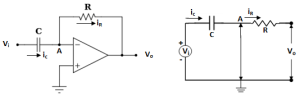Fig.5 (i)                                                                              Fig .5 (ii)

It consists of an OP-Amp, an input capacitor C and feedback resistor R.

It can be noted that the placement of the capacitor and resistor differs from the integrator circuit.

#### Circuit Analysis

Since point A in fig.5 (i) is at virtual ground, the virtual-ground equivalent circuit of the operational differentiator will be as shown in fig.5 (ii).

Because of virtual ground and infinite impedance of OP-Amp, all the input current ic flows through the feedback resistor R.

i.e.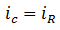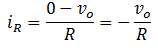And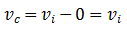Also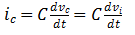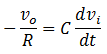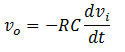The above equation shows that output is the differentiation of the input with an inversion and scale multiplier of RC.

If the input voltage is constant, dvi/dt is zero and the output voltage is zero.

The faster the input voltage changes, the larger the magnitude of the output voltage.

Comparator

Often we want to compare one voltage to another to see which is larger. In this situation, a comparator may be used.

A comparator is an OP-Amp circuit without negative feedback and takes advantage of very high open-loop voltage gain.

A comparator has two input voltages and one output voltage.

Because of the high open loop voltage gain of an OP-Amp a very small difference voltage between the two inputs drives the amplifier to saturation. This is the key point in the working of comparator.

Fig.6 shows the action of a comparator. The input voltages are v1(signal) & v2(reference voltage).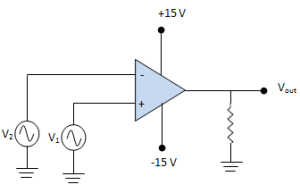Fig.6

If the differential input is positive, the circuit is driven to saturation & output goes to maximum positive value.

Reverse happens, when the differential input goes negative i.e. now output is maximum negative.

This circuit is called comparator because it compares v1 to v2 to produce a saturated positive or negative output voltage.

#### Comparator Circuits

A comparator circuit has the following two characteristics:

1. It uses no feedback so that the voltage gain is equal to the open loop voltage gain of OP-Amp.
2. It is operated in a non-linear mode

#### 1.  As a square wave generator:

A comparator can be used to produce a square wave output from a sine wave input.

Fig.7 shows the circuit of a comparator to produce square wave output.Fig.7

It can be noted that the inverting terminal is grounded and signal vin is applied to the non-inverting terminal.

Since the gain of a comparator is equal to AOL , virtually any difference voltage at the inputs will cause the output to go to one of the saturation stage and stay there until the voltage difference is removed.

The polarity of the input difference voltage will determine to which saturation level the output of the comparator goes.

When the input signal goes positive, the output jumps to about to +13 V. When the input goes negative, the output jumps to about -13 V.

The output changes rapidly, from -13 V to +13 V and vice-versa. This change is so rapid that we get a square wave output for a sine wave input.

#### 2. As a zero crossing detector:

When one input of a comparator is connected to ground, it is known as zero crossing detector because the output changes when the input crosses 0 V.

The zero crossing circuit is shown in fig.8 with its input and output wave forms.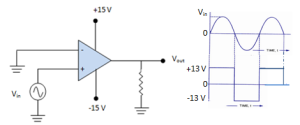Fig.8 (i)                                               Fig.8 (ii)

When the input signal is positive-going, the output is driven to positive maximum value. When the input crosses the zero axis and begins to go negative, the output is driven to negative maximum value.

3.  As a level detector :

When a comparator is used to compare a signal amplitude to a fixed d.c. level (reference voltage), the circuit is known as a level detector.

We can modify zero crossing detector circuit to construct a level detector by connecting a fixed reference voltage VREF  to the inverting input of the OP-Amp as shown in fig.9(i).A more practical arrangement is shown in fig.9(ii) using a voltage divider to set the reference voltage as follows: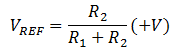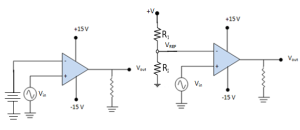Fig.9(i)                                                           Fig.9(ii)

#### Circuit Action

Suppose the input vin  is a sine wave. When the input voltage is less than the reference voltage, the output goes to maximum negative level. It remains here until vin increases above VREF .

When the input voltage exceeds the reference voltage, the out goes to its maximum positive state. It remains here until vin decreases below VREF . This circuit is used for non zero-level detection. Fig.10 shows the input /output waveforms.Fig.10